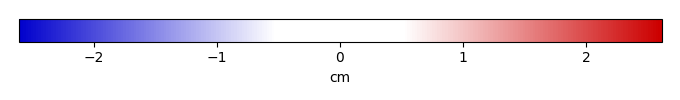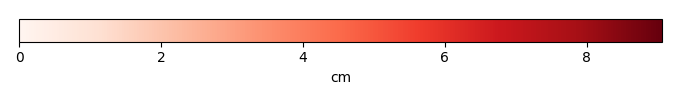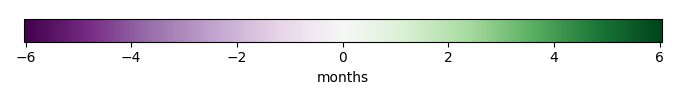# Mean State

Period Mean (original grids) [cm]
Model Period Mean (intersection) [cm]
Model Period Mean (complement) [cm]
Benchmark Period Mean (intersection) [cm]
Benchmark Period Mean (complement) [cm]
Bias [cm]
RMSE [cm]
Phase Shift [months]
Bias Score 
RMSE Score 
Seasonal Cycle Score 
Spatial Distribution Score 
Interannual Variability Score 
Overall Score 
Benchmark [-] 1.16e-07
CLM5PHSOFF [-] 0.00 0.00 0.00 1.13e-07 0.00 -1.05e-07 2.15e-06 4.77 0.95 0.37 0.12 0.37
CLM5PHSON [-] 0.00 0.00 0.00 1.13e-07 0.00 -1.05e-07 2.15e-06 4.77 0.95 0.37 0.12 0.37
Period Mean (original grids) [cm]
Model Period Mean (intersection) [cm]
Model Period Mean (complement) [cm]
Benchmark Period Mean (intersection) [cm]
Benchmark Period Mean (complement) [cm]
Bias [cm]
RMSE [cm]
Phase Shift [months]
Bias Score 
RMSE Score 
Seasonal Cycle Score 
Spatial Distribution Score 
Interannual Variability Score 
Overall Score 
Benchmark [-] 5.23e-07
CLM5PHSOFF [-] 1.86e-08 0.00 2.45e-08 4.98e-07 0.00 -5.09e-07 6.32e-06 4.49 0.93 0.37 0.20 0.37
CLM5PHSON [-] 3.08e-09 0.00 4.08e-09 4.98e-07 0.00 -5.09e-07 6.32e-06 4.49 0.93 0.37 0.20 0.37
Period Mean (original grids) [cm]
Model Period Mean (intersection) [cm]
Model Period Mean (complement) [cm]
Benchmark Period Mean (intersection) [cm]
Benchmark Period Mean (complement) [cm]
Bias [cm]
RMSE [cm]
Phase Shift [months]
Bias Score 
RMSE Score 
Seasonal Cycle Score 
Spatial Distribution Score 
Interannual Variability Score 
Overall Score 
Benchmark [-] 4.86
CLM5PHSOFF [-] 16.2 8.60 5.40 4.84 0.00 3.73 6.46 0.386 0.56 0.45 0.97 0.60 0.44 0.58
CLM5PHSON [-] 8.06 7.99 9.35 4.84 0.00 3.26 6.10 0.340 0.59 0.47 0.97 0.61 0.46 0.59
Period Mean (original grids) [cm]
Model Period Mean (intersection) [cm]
Model Period Mean (complement) [cm]
Benchmark Period Mean (intersection) [cm]
Benchmark Period Mean (complement) [cm]
Bias [cm]
RMSE [cm]
Phase Shift [months]
Bias Score 
RMSE Score 
Seasonal Cycle Score 
Spatial Distribution Score 
Interannual Variability Score 
Overall Score 
Benchmark [-] 3.74
CLM5PHSOFF [-] 4.88 4.90 3.33 3.59 0.00 1.30 2.87 0.376 0.71 0.57 0.97 0.84 0.62 0.72
CLM5PHSON [-] 4.72 4.72 6.73 3.59 0.00 1.12 2.84 0.375 0.72 0.58 0.97 0.83 0.62 0.72
Period Mean (original grids) [cm]
Model Period Mean (intersection) [cm]
Model Period Mean (complement) [cm]
Benchmark Period Mean (intersection) [cm]
Benchmark Period Mean (complement) [cm]
Bias [cm]
RMSE [cm]
Phase Shift [months]
Bias Score 
RMSE Score 
Seasonal Cycle Score 
Spatial Distribution Score 
Interannual Variability Score 
Overall Score 
Benchmark [-] 0.616
CLM5PHSOFF [-] 0.784 0.791 0.644 0.558 0.00 0.239 1.00 0.520 0.67 0.36 0.95 0.77 0.49 0.60
CLM5PHSON [-] 0.831 0.828 1.00 0.558 0.00 0.248 1.09 0.563 0.66 0.36 0.95 0.76 0.49 0.60
Period Mean (original grids) [cm]
Model Period Mean (intersection) [cm]
Model Period Mean (complement) [cm]
Benchmark Period Mean (intersection) [cm]
Benchmark Period Mean (complement) [cm]
Bias [cm]
RMSE [cm]
Phase Shift [months]
Bias Score 
RMSE Score 
Seasonal Cycle Score 
Spatial Distribution Score 
Interannual Variability Score 
Overall Score 
Benchmark [-] 0.114
CLM5PHSOFF [-] 0.135 0.127 0.137 0.119 0.00 0.0183 0.309 1.33 0.83 0.36 0.80 0.67 0.37 0.56
CLM5PHSON [-] 0.114 0.0872 0.131 0.119 0.00 -0.0217 0.274 1.36 0.84 0.36 0.80 0.77 0.37 0.58
Period Mean (original grids) [cm]
Model Period Mean (intersection) [cm]
Model Period Mean (complement) [cm]
Benchmark Period Mean (intersection) [cm]
Benchmark Period Mean (complement) [cm]
Bias [cm]
RMSE [cm]
Phase Shift [months]
Bias Score 
RMSE Score 
Seasonal Cycle Score 
Spatial Distribution Score 
Interannual Variability Score 
Overall Score 
Benchmark [-] 5.87
CLM5PHSOFF [-] 10.8 11.0 8.04 5.97 0.00 5.11 7.58 0.460 0.48 0.43 0.96 0.63 0.39 0.55
CLM5PHSON [-] 11.2 11.2 14.6 5.97 0.00 5.24 7.86 0.545 0.48 0.42 0.95 0.42 0.38 0.51
Period Mean (original grids) [cm]
Model Period Mean (intersection) [cm]
Model Period Mean (complement) [cm]
Benchmark Period Mean (intersection) [cm]
Benchmark Period Mean (complement) [cm]
Bias [cm]
RMSE [cm]
Phase Shift [months]
Bias Score 
RMSE Score 
Seasonal Cycle Score 
Spatial Distribution Score 
Interannual Variability Score 
Overall Score 
Benchmark [-] 0.906
CLM5PHSOFF [-] 2.46 2.04 6.28 0.893 0.00 1.21 2.36 0.355 0.56 0.25 0.98 0.42 0.33 0.46
CLM5PHSON [-] 2.78 2.39 10.5 0.893 0.00 1.22 2.52 0.389 0.54 0.24 0.97 0.47 0.32 0.46
Period Mean (original grids) [cm]
Model Period Mean (intersection) [cm]
Model Period Mean (complement) [cm]
Benchmark Period Mean (intersection) [cm]
Benchmark Period Mean (complement) [cm]
Bias [cm]
RMSE [cm]
Phase Shift [months]
Bias Score 
RMSE Score 
Seasonal Cycle Score 
Spatial Distribution Score 
Interannual Variability Score 
Overall Score 
Benchmark [-] 1.61
CLM5PHSOFF [-] -8.81e+15 2.82 0.512 1.61 0.00 1.22 2.39 0.821 0.67 0.37 0.90 0.59 0.44 0.56
CLM5PHSON [-] 2.57 2.79 1.97 1.61 0.00 1.20 2.49 0.861 0.66 0.37 0.90 0.51 0.44 0.54
Period Mean (original grids) [cm]
Model Period Mean (intersection) [cm]
Model Period Mean (complement) [cm]
Benchmark Period Mean (intersection) [cm]
Benchmark Period Mean (complement) [cm]
Bias [cm]
RMSE [cm]
Phase Shift [months]
Bias Score 
RMSE Score 
Seasonal Cycle Score 
Spatial Distribution Score 
Interannual Variability Score 
Overall Score 
Benchmark [-] 0.0943
CLM5PHSOFF [-] 0.0606 0.0860 0.00745 0.0885 0.00 -0.0118 0.185 1.65 0.87 0.36 0.74 0.84 0.41 0.60
CLM5PHSON [-] 0.0433 0.0572 0.00676 0.0885 0.00 -0.0385 0.166 1.66 0.87 0.36 0.74 0.76 0.41 0.58
Period Mean (original grids) [cm]
Model Period Mean (intersection) [cm]
Model Period Mean (complement) [cm]
Benchmark Period Mean (intersection) [cm]
Benchmark Period Mean (complement) [cm]
Bias [cm]
RMSE [cm]
Phase Shift [months]
Bias Score 
RMSE Score 
Seasonal Cycle Score 
Spatial Distribution Score 
Interannual Variability Score 
Overall Score 
Benchmark [-] 3.40
CLM5PHSOFF [-] 8.52 7.77 11.8 3.47 0.00 4.36 6.69 0.904 0.39 0.32 0.92 0.40 0.30 0.44
CLM5PHSON [-] 8.33 7.75 12.0 3.47 0.00 4.58 7.12 0.979 0.39 0.31 0.91 0.21 0.30 0.41
Period Mean (original grids) [cm]
Model Period Mean (intersection) [cm]
Model Period Mean (complement) [cm]
Benchmark Period Mean (intersection) [cm]
Benchmark Period Mean (complement) [cm]
Bias [cm]
RMSE [cm]
Phase Shift [months]
Bias Score 
RMSE Score 
Seasonal Cycle Score 
Spatial Distribution Score 
Interannual Variability Score 
Overall Score 
Benchmark [-] 0.000681
CLM5PHSOFF [-] 1.76e-05 5.07e-05 2.64e-08 0.000651 0.00 -0.000588 0.00299 0.796 0.86 0.37 0.91 0.036 0.41 0.49
CLM5PHSON [-] 1.52e-05 2.96e-05 3.23e-08 0.000651 0.00 -0.000611 0.00301 0.807 0.86 0.37 0.91 0.011 0.39 0.48
Period Mean (original grids) [cm]
Model Period Mean (intersection) [cm]
Model Period Mean (complement) [cm]
Benchmark Period Mean (intersection) [cm]
Benchmark Period Mean (complement) [cm]
Bias [cm]
RMSE [cm]
Phase Shift [months]
Bias Score 
RMSE Score 
Seasonal Cycle Score 
Spatial Distribution Score 
Interannual Variability Score 
Overall Score 
Benchmark [-] 0.302
CLM5PHSOFF [-] 0.651 0.678 0.108 0.265 0.00 0.444 1.06 0.437 0.66 0.26 0.97 0.40 0.38 0.49
CLM5PHSON [-] 0.810 0.810 1.09 0.265 0.00 0.524 1.28 0.567 0.63 0.24 0.96 0.36 0.34 0.46
Period Mean (original grids) [cm]
Model Period Mean (intersection) [cm]
Model Period Mean (complement) [cm]
Benchmark Period Mean (intersection) [cm]
Benchmark Period Mean (complement) [cm]
Bias [cm]
RMSE [cm]
Phase Shift [months]
Bias Score 
RMSE Score 
Seasonal Cycle Score 
Spatial Distribution Score 
Interannual Variability Score 
Overall Score 
Benchmark [-] 3.28
CLM5PHSOFF [-] 5.98 5.11 20.3 3.10 0.00 2.08 4.65 0.573 0.55 0.39 0.96 0.67 0.49 0.58
CLM5PHSON [-] 5.89 5.28 18.1 3.10 0.00 2.11 5.14 0.700 0.54 0.37 0.95 0.81 0.46 0.58
Period Mean (original grids) [cm]
Model Period Mean (intersection) [cm]
Model Period Mean (complement) [cm]
Benchmark Period Mean (intersection) [cm]
Benchmark Period Mean (complement) [cm]
Bias [cm]
RMSE [cm]
Phase Shift [months]
Bias Score 
RMSE Score 
Seasonal Cycle Score 
Spatial Distribution Score 
Interannual Variability Score 
Overall Score 
Benchmark [-] 0.619
CLM5PHSOFF [-] 0.712 0.718 0.0592 0.579 0.00 0.141 1.06 0.535 0.73 0.37 0.96 0.63 0.49 0.59
CLM5PHSON [-] 0.559 0.559 0.200 0.579 0.00 -0.0315 1.01 0.563 0.73 0.37 0.96 0.75 0.50 0.62
Period Mean (original grids) [cm]
Model Period Mean (intersection) [cm]
Model Period Mean (complement) [cm]
Benchmark Period Mean (intersection) [cm]
Benchmark Period Mean (complement) [cm]
Bias [cm]
RMSE [cm]
Phase Shift [months]
Bias Score 
RMSE Score 
Seasonal Cycle Score 
Spatial Distribution Score 
Interannual Variability Score 
Overall Score 
Benchmark [-] 1.54e-05
CLM5PHSOFF [-] 0.00 0.00 0.00 1.54e-05 0.00 -1.54e-05 0.000214 2.05 0.93 0.37 0.75 0.37
CLM5PHSON [-] 0.00 0.00 0.00 1.54e-05 0.00 -1.54e-05 0.000214 2.05 0.93 0.37 0.75 0.37

# Temporally integrated period mean

BENCHMARK MEANMODEL MEANBIASBIAS SCORERMSERMSE SCOREBENCHMARK INTERANNUAL VARIABILITYMODEL INTERANNUAL VARIABILITYINTERANNUAL VARIABILITY SCOREBENCHMARK MAX MONTHMODEL MAX MONTHDIFFERENCE IN MAX MONTHSEASONAL CYCLE SCORESPATIAL TAYLOR DIAGRAMMODEL COLORS# Spatially integrated regional mean

MODEL COLORSREGIONAL MEANANNUAL CYCLEMONTHLY ANOMALYANNUAL CYCLE# All Models

BenchmarkCLM5PHSOFFCLM5PHSON